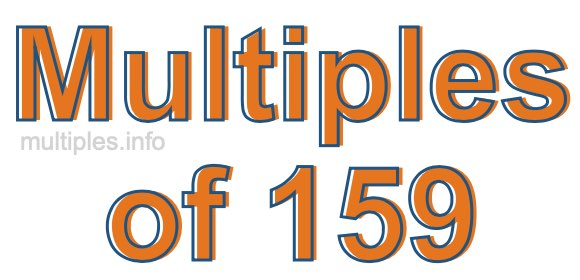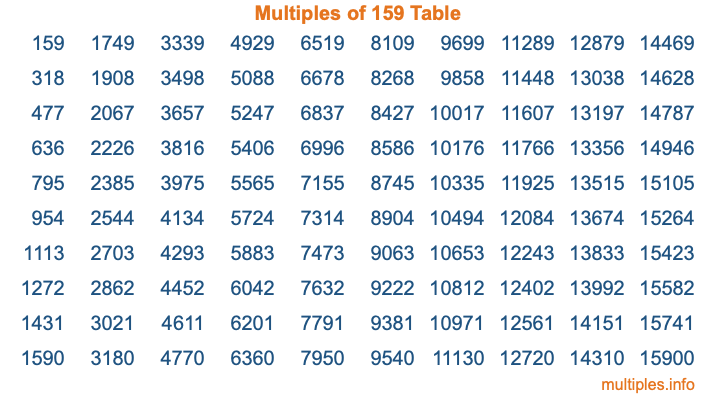Multiples of 159Welcome to the Multiples of 159 page. Here we will first teach you everything you will ever need to know about the multiples of 159, and then give you a study guide summary of everything we taught you to make sure you remember it all. Use this page to look up facts and learn information about the multiples of 159. This page will make you a multiples of one hundred fifty-nine expert!

Definition of Multiples of 159
Multiples of 159 are all the numbers that when divided by 159 equal an integer. Each of the multiples of 159 are called a multiple. A multiple of 159 is created by multiplying 159 by an integer.

Therefore, to create a list of multiples of 159, you start with 1 multiplied by 159, then 2 multiplied by 159, then 3 multiplied by 159, and so on for as long as you want. Thus, the list of the first five multiples of 159 is 159, 318, 477, 636, and 795. To see a larger list of multiples of 159, see the printable image of Multiples of 159 further down on this page. We also have a category where you can choose any nth multiple of 159.

Multiples of 159 Checker
The Multiples of 159 Checker below checks to see if any number of your choice is a multiple of 159. In other words, it checks to see if there is any number (integer) that when multiplied by 159 will equal your number. To do that, we divide your number by 159. If the the quotient is an integer, then your number is a multiple of 159.

Is  a multiple of 159?

Least Common Multiple of 159 and ...
A Least Common Multiple (LCM) is the lowest multiple that two or more numbers have in common. This is also called the smallest common multiple or lowest common multiple and is useful to know when you are adding our subtracting fractions. Enter one or more numbers below (159 is already entered) to find the LCM.

Check out our LCM Calculator if you need more details about the Least Common Multiple or if you need the LCM for different numbers for adding and subtraction fractions.

nth Multiple of 159
As we stated above, 159 is the first multiple of 159, 318 is the second multiple of 159, 477 is the third multiple of 159, and so on. Enter a number below to find the nth multiple of 159.

th multiple of 159

Multiples of 159 vs Factors of 159
159 is a multiple of 159 and a factor of 159, but that is where the similarities end. All postive multiples of 159 are 159 or greater than 159. All positive factors of 159 are 159 or less than 159.

Below is the beginning list of multiples of 159 and the factors of 159 so you can compare:

Multiples of 159: 159, 318, 477, 636, 795, etc.

Factors of 159: 1, 3, 53, 159

As you can see, the multiples of 159 are all the numbers that you can divide by 159 to get a whole number. The factors of 159, on the other hand, are all the whole numbers that you can multiply by another whole number to get 159.

It's also interesting to note that if a number (x) is a factor of 159, then 159 will also be a multiple of that number (x).

Multiples of 159 vs Divisors of 159
The divisors of 159 are all the integers that 159 can be divided by evenly. Below is a list of the divisors of 159.

Divisors of 159: 1, 3, 53, 159

The interesting thing to note here is that if you take any multiple of 159 and divide it by a divisor of 159, you will see that the quotient is an integer.

Multiples of 159 Table
Below is an image of the first 100 multiples of 159 in a table. The table is in chronological order, column by column. The first column has the first ten multiples of 159, the second column has the next ten multiples of 159, and so on.The Multiples of 159 Table is also referred to as the 159 Times Table or Times Table of 159. You are welcome to print out our table for your studies.

Negative Multiples of 159
Although not often discussed or needed in math, it is worth mentioning that you can make a list of negative multiples of 159 by multiplying 159 by -1, then by -2, then by -3, and so on, to get the following list of negative multiples of 159:

-159, -318, -477, -636, -795, etc.

Multiples of 159 Summary
Below is a summary of important Multiples of 159 facts that we have discussed on this page. To retain the knowledge on this page, we recommend that you read through the summary and explain to yourself or a study partner why they hold true.

There are an infinite number of multiples of 159.

A multiple of 159 divided by 159 will equal a whole number.

159 divided by a factor of 159 equals a divisor of 159.

The nth multiple of 159 is n times 159.

The largest factor of 159 is equal to the first positive multiple of 159.

159 is a multiple of every factor of 159.

159 is a multiple of 159.

A multiple of 159 divided by a divisor of 159 equals an integer.

159 divided by a divisor of 159 equals a factor of 159.

Any integer times 159 will equal a multiple of 159.

Multiples of a Number
Here you can get the multiples of another number, all with the same attention to detail as we did for multiples of 159 on this page.

Multiples of
Multiples of 160
Did you find our page about multiples of one hundred fifty-nine educational? Do you want more knowledge? Check out the multiples of the next number on our list!